# Thermodynamics - 1

## 15 Questions MCQ Test GATE Mechanical (ME) 2023 Mock Test Series | Thermodynamics - 1

Description
Attempt Thermodynamics - 1 | 15 questions in 45 minutes | Mock test for GATE preparation | Free important questions MCQ to study GATE Mechanical (ME) 2023 Mock Test Series for GATE Exam | Download free PDF with solutions
QUESTION: 1

### Consider the following properties: Temperature Viscosity Specific entropy Thermal conductivity Which of the above properties of a system is/are intensive?

Solution:

(d) Intensive properties are independent of the mass in the system, for example, density, pressure, temperature. Extensive properties are related to mass, for example, volume, energy.

QUESTION: 2

### Pressure reaches a value of absolute zero

Solution:

(d) Temperature and pressure are the measures of molecular moment of the system. The molecular moment of the system is zero, the absolute temperature and absolute pressure becomes zero.

QUESTION: 3

### Ice kept in a well insulated thermos flask is an example of which system?

Solution:

(b) Heat and work cannot be transferred into the ice kept in a well insulated thermos flask, hence, it is an isolated system.

QUESTION: 4

A thermodynamic system is considered to be an isolated one if

Solution:

(c) An isolated system has no interaction with its surroundings.

QUESTION: 5

The phenomenon of magnetic arc blow is observed almost exclusively with

Solution:
QUESTION: 6

Work transfer between the system and surround­ings

Solution:

(d) The change in a property of a system is independent of the path the system follows during the change of state. Therefore, properties are point functions. Work transfer is not a property of the system.

QUESTION: 7

A hot metal piece kept in air cools from 80°C to 70°C in t1 seconds, from 70°C to 60°C in t2 seconds and from 60°C to 50°C in t3 seconds, then

Solution:
QUESTION: 8

A cyclic heat engine does 50 kJ of work per cycle. If the efficiency of the heat engine is 75%, the heat rejected per cycle is

Solution:

Given that

W = 50 KJ

η = 0.75

Heat rejected per cycle is determned as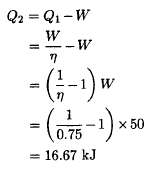QUESTION: 9

It is proposed to build a refrigeration plant for cold storage to be maintained at — 3°C. The ambient temperature is 27° C. If 5 x 106 kJ/h of energy is tobe continuously removed from the cold storage, the minimum power required to run the refrigerator will be

Solution:

Given that

T2 = 270 K

T1 = 27 + 273

=  300 K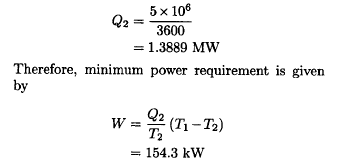QUESTION: 10

A reversible engine has ideal thermal efficiency of 30%. When it is used as a refrigeration machine with all other conditions unchanged, the coefficient of performance will be

Solution:

Given that ηe = 0.3 . The COP of the refrigerater is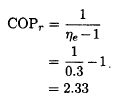QUESTION: 11

A heat engine is supplied with 250 kJ/s of heat at a constant fixed temperature of 227° C. The heat is rejected at 27° C. The cycle is reversible, if the amount of heat rejected is

Solution:

The  minimum heat rejected by reversible cycle is given by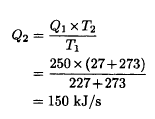QUESTION: 12

Which one of the following pairs best expresses a relationship similar to that expressed in the pair “pressure-volume” for a thermodynamic system undergoing a process?

Solution:
QUESTION: 13

A heat pump working on a reversed Carnot cycle has a COP of 5. If it works as a refrigerator taking 1 kW of work input, the refrigeration effect will be

Solution:

COP of the heat Pump is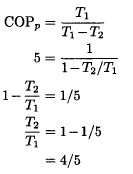The COP of the refrigerater is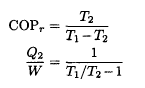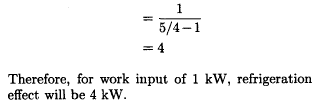QUESTION: 14

One reversible heat engine operates between 1600 K and T2 K, and smother reversible engine operates between T2 K and 400 K. If both engines have the same heat input and output, then the temperature T2 must be equal to

Solution:

The given condition means equal efficiency of both engines , therefore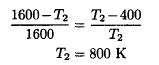QUESTION: 15

A heat engine using lake water at 12° C as source and the surrounding atmosphere at 2°C as sink executes 1080 cycles per minute. If the amount of heat drawn per cycle is 57 J, then the output of the engine will be

Solution:

Given That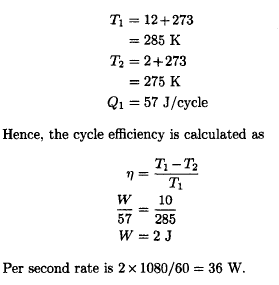Use Code STAYHOME200 and get INR 200 additional OFF Use Coupon Code